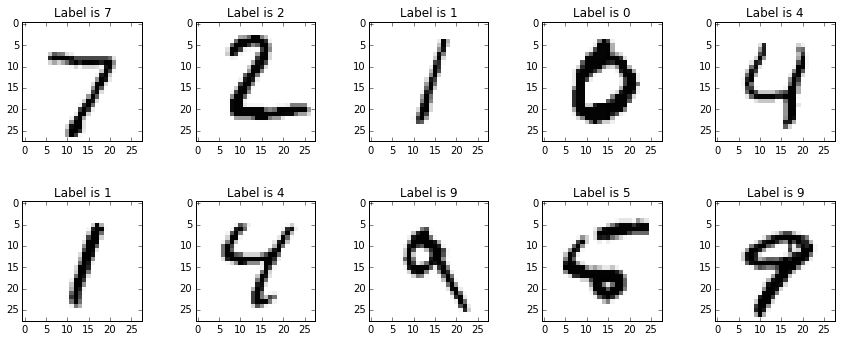## Sunday, 1 March 2015

### The MNIST Dataset of Handwitten Digits

In the machine learning community common data sets have emerged. Having common datasets is a good way of making sure that different ideas can be tested and compared in a meaningful way - because the data they are tested against is the same.

You may have come across the famous iris flower dataset which is very common for clustering or classification algorithms.

With our neural network, we eventually want it to classify human handwritten numbers. So we'd want to train it on a dataset of handwritten numbers, with labels to tell us what the numbers should be. There is in fact a very popular such dataset called the MNIST dataset. It's a big database, with 60,000 training examples, and 10,000 for testing.

The format of the MNIST database isn't the easiest to work with, so others have created simpler CSV files, such as this one. The CSV files are:

The format of these is easy to understand:

• The first value is the "label", that is, the actual digit that the handwriting is supposed to represent, such as a "7" or a "9". It is the answer to which the neural network is aspiring to classify.
• The subsequent values, all comma separated, are the pixel values of the handwritten digit. The size of the pixel array is 28 by 28, so there are 784 values after the label.

These files are still big, and it would be nicer to work with much smaller ones whilst we experiment and develop our code for visualising handwriting and the neural networks themselves.

So here are smaller versions of the above CSV files, but with 100 training and 10 test items:
Next we'll try to load these files into python and see if we can display the handwritten characters.

We can load the data easily from a file as follows:

f = open("mnist_test_10.csv", 'r')
f.close()

We can then split each line according the commas, to get the label and the bitmap values, from which we build an array. We do need to reshape the linear array to 28x28 before we display it.

f = figure(figsize=(15,15));
count=1
for line in a:
linebits = line.split(',')
imarray = numpy.asfarray(linebits[1:]).reshape((28,28))
subplot(5,5,count)
count += 1
title("Label is " + linebits)
imshow(imarray, cmap='Greys', interpolation='None')
pass

The output in IPython is a series of images, You can check that the label matches the handwritten image:Cool - we can now import handwritten image data from the MNIST dataset and work with it in Python!

PS Yes, the "subplots" command for each loop isn't efficient but I didnt' have time to work out how to do plotting subplots properly.

UPDATE: The book is out! - and provides examples of working with the MNIST data set, as well as using your own handwriting to create a test dataset.